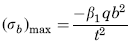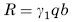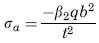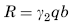Related Resources: calculators

### Loading Uniformly decreasing from fixed edge to simply supported edge Stress and Deflection Equatio

Flat Rectangular Plate, Three Edges Fixed, One Edge (a) Simply Supported Loading Uniformly decreasing from fixed edge to simply supported edge Stress and Deflection Equation and Calculator.

Per. Roarks Formulas for Stress and Strain for flat plates with straight boundaries and constant thickness

Flat Rectangular plate, Three Edges Fixed, One Edge Load Uniformly Decreasing from Fixed Edge to Simply Supported Edge Plate

 Rectangular plate; Three Edges FixedLoad uniformly decreasing from fixed edge to simply supported edgeAt x = 0, z = 0
StressReaction ForceStress at x = ±a/2, z = 0.4bReaction ForceWhere used:
E = Modulus of Elasticity (lbs/in2)
q = Total load or force to Plate (lbs/in2)
v = Poisson’s ratio (assumed to be 0.3)
t = plate thickness, (in)
a = plate length, (in)
b = plate width, (in)
σb = stress, (lbs/in2)
R = Reaction Force (lbs/in)
β1,2 = Constant From Table A
γ1,2 = Constant From Table A

Table A

 a/b 0.25 0.5 0.75 1 1.5 2 3 β1 0.018 0.064 0.12 0.192 0.303 0.356 0.382 β2 0.019 0.068 0.124 0.161 0.181 0.168 0.132 γ1 0.106 0.195 0.265 0.323 0.383 0.399 0.4 γ2 0.075 0.152 0.212 0.245 0.262 0.258 0.25

Reference:

Roarks Formulas for Stress and Strain, 7th Edition